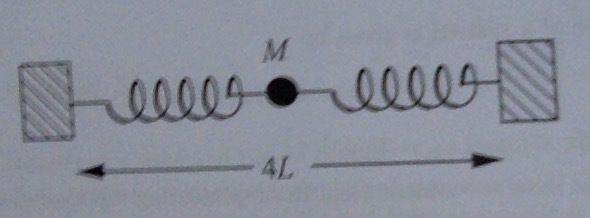# Transverse Oscillation of horizontal Mass-Springs system

## Homework Statement

Mass M is supported by a smooth table and connected by two light horizontal springs to two fixed blocks. Each spring is of natural length L. Both springs are initially extended by L to get a total width between blocks of 4L. The spring constant of both springs is k.

When the mass is given a small transverse displacement, y, show that the restoring force is approximately ky.

See photo below:F = kx
sinθ = o/h[/B]

## The Attempt at a Solution

Restoring force, Fr = 2kxsinθ
sinθ = y / (2L + x)
x = (y / sinθ) - 2L

Chandra Prayaga
First you should draw a diagram showing a small transverse displacement y of the ball, and draw a coordinate system. Then write your equations. For example, I don't understand why there is an x in your equations at all.

First you should draw a diagram showing a small transverse displacement y of the ball, and draw a coordinate system. Then write your equations. For example, I don't understand why there is an x in your equations at all.

The x is to do with the extension of the spring which will occur when you displace the ball by y upwards.

haruspex
Homework Helper
Gold Member
2020 Award
Restoring force, Fr = 2kxsinθ
sinθ = y / (2L + x)
Are you being consistent about the meaning of x? Is it the total extension of the spring, or the additional extension beyond its extension when y=0?

Are you being consistent about the meaning of x? Is it the total extension of the spring, or the additional extension beyond its extension when y=0?

Additional extension beyond what it was (L) at y=0.

haruspex
Homework Helper
Gold Member
2020 Award
Additional extension beyond what it was (L) at y=0.
Then reconsider your equation for Fr.

Then reconsider your equation for Fr.

Ah, good point.

So it should be: Fr = 2k(L+x)sinθ

Which would then be: = 2ky(L+x) / (2L+x)

Agreed?

haruspex
Homework Helper
Gold Member
2020 Award
Ah, good point.

So it should be: Fr = 2k(L+x)sinθ

Which would then be: = 2ky(L+x) / (2L+x)

Agreed?
Yes.

Yes.

I'm still unsure how to get from there to simply ky however.

haruspex
Homework Helper
Gold Member
2020 Award
I'm still unsure how to get from there to simply ky however.
It says small displacements. If y is small, x is very small. What approximations does that allow you to make?

It says small displacements. If y is small, x is very small. What approximations does that allow you to make?

Expanding previously, Fr = (2kL+2kx)y / (2L+x), so if x is small then 2kx ≈ 0 and 2L + x ≈ 2L? Which would leave 2kLy/2L = ky but then surely the y on the top would make the whole thing ≈ 0?

haruspex
Homework Helper
Gold Member
2020 Award
Expanding previously, Fr = (2kL+2kx)y / (2L+x), so if x is small then 2kx ≈ 0 and 2L + x ≈ 2L? Which would leave 2kLy/2L = ky but then surely the y on the top would make the whole thing ≈ 0?
What we're doing here is finding the first order approximation. You can think of it as writing the full form as the sum of a series of terms in which, as the displacement tends to zero, each term tends to zero faster than the one before it: a power series, say. The first order approximation throws away all except the first term.

•omegasquared
Chandra Prayaga
•